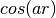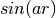# matminer.featurizers.utils package¶

## matminer.featurizers.utils.cgcnn module¶

class `matminer.featurizers.utils.cgcnn.``AtomCustomArrayInitializer`(**kwargs)

Bases: `object`

Initialize atom feature vectors using a JSON file, which is a python dictionary mapping from element number to a list representing the feature vector of the element.

Args:

elem_embedding_file (str): The path to the .json file

`__init__`(elem_embedding)

Initialize self. See help(type(self)) for accurate signature.

class `matminer.featurizers.utils.cgcnn.``CIFDataWrapper`(**kwargs)

Bases: `object`

Wrapper for a dataset containing pymatgen Structure objects. This is modified from CGCNN repo’s CIFData for wrapping dataset where the structures are stored in CIF files. As we already have X as an iterable of pymatgen Structure objects, we can use this wrapper instead of CIFData.

`__init__`(X, y, atom_init_fea, max_num_nbr=12, radius=8, dmin=0, step=0.2, random_seed=123)
Args:

X (Series/list): An iterable of pymatgen Structure objects. y (Series/list): target property that CGCNN is to predict. atom_init_fea (dict): A dict of {atom type: atom feature}. max_num_nbr (int): The max number of every atom’s neighbors. radius (float): Cutoff radius for searching neighbors. dmin (int): The minimum distance for constructing GaussianDistance. step (float): The step size for constructing GaussianDistance. random_seed (int): Random seed for shuffling the dataset.

class `matminer.featurizers.utils.cgcnn.``CrystalGraphConvNetWrapper`(**kwargs)

Bases: `object`

Wrapper for CrystalGraphConvNet in the CGCNN repo and add extract_feature function to extract the feature vector after pooling layer of CGCNN model as features for the structures. Please see the CrystalGraphConvNet in the CGCNN repo for more details

`__init__`(orig_atom_fea_len, nbr_fea_len, atom_fea_len=64, n_conv=3, h_fea_len=128, n_h=1, classification=False)
Args:

orig_atom_fea_len (int): Number of atom features in the input. nbr_fea_len (int): Number of bond features. atom_fea_len (int): Number of hidden atom features

in the convolutional layers.

n_conv (int): Number of convolutional layers. h_fea_len (int): Number of hidden features after pooling. n_h (int): Number of hidden layers after pooling. classification (bool): Classification task or regression task.

`extract_feature`(atom_fea, nbr_fea, nbr_fea_idx, crystal_atom_idx)

Extract the feature vector after pooling layer of CGCNN model as features for the structures.

Args:
atom_fea (Variable(torch.Tensor)): shape (N, orig_atom_fea_len)

Atom features from atom type.

nbr_fea (Variable(torch.Tensor)): shape (N, M, nbr_fea_len)

Bond features of each atom’s M neighbors.

nbr_fea_idx (torch.LongTensor): shape (N, M)

Indices of M neighbors of each atom.

crystal_atom_idx (list of torch.LongTensor): length N0

Mapping from the crystal idx to atom idx.

Returns:

feature (list): deep learning feature

`matminer.featurizers.utils.cgcnn.``appropriate_kwargs`(kwargs, func)

Auto get the appropriate kwargs according to those allowed by the func. Args:

kwargs (dict): kwargs. func (object): function object.

Returns:

filtered_dict (dict): filtered kwargs.

## matminer.featurizers.utils.grdf module¶

Functions designed to work with General Radial Distribution Function

class `matminer.featurizers.utils.grdf.``AbstractPairwise`

Bases: `object`

Abstract class for pairwise functions used in Generalized Radial Distribution Function

`name`()

Make a label for this pairwise function

Returns:

(string) Label for the function

`volume`(cutoff)

Compute the volume of this pairwise function

Args:

cutoff (float): Cutoff distance for radial distribution function

Returns:

(float): Volume of bin

class `matminer.featurizers.utils.grdf.``Bessel`(n)

Bessel pairwise function

`__init__`(n)

Initialize the function

Args:

n (int): Degree of Bessel function

class `matminer.featurizers.utils.grdf.``Cosine`(a)

Cosine pairwise function:`__init__`(a)

Initialize the function

Args:

a (float): Frequency factor for cosine function

`volume`(cutoff)

Compute the volume of this pairwise function

Args:

cutoff (float): Cutoff distance for radial distribution function

Returns:

(float): Volume of bin

class `matminer.featurizers.utils.grdf.``Gaussian`(width, center)

Gaussian function, with specified width and center

`__init__`(width, center)

Initialize the gaussian function

Args:

width (float): Width of the gaussian center (float): Center of the gaussian

`volume`(cutoff)

Compute the volume of this pairwise function

Args:

cutoff (float): Cutoff distance for radial distribution function

Returns:

(float): Volume of bin

class `matminer.featurizers.utils.grdf.``Histogram`(start, width)

Rectangular window function, used in conventional Radial Distribution Functions

`__init__`(start, width)

Initialize the window function

Args:

start (float): Beginning of window width (float): Size of window

`volume`(cutoff)

Compute the volume of this pairwise function

Args:

cutoff (float): Cutoff distance for radial distribution function

Returns:

(float): Volume of bin

class `matminer.featurizers.utils.grdf.``Sine`(a)

Sine pairwise function:`__init__`(a)

Initialize the function

Args:

a (float): Frequency factor for sine function

`volume`(cutoff)

Compute the volume of this pairwise function

Args:

cutoff (float): Cutoff distance for radial distribution function

Returns:

(float): Volume of bin

`matminer.featurizers.utils.grdf.``initialize_pairwise_function`(name, **options)

Create a new pairwise function object

Args:

name (string): Name of class to instantiate

Keyword Arguments:

Any options for the pairwise class (see each pairwise function for details)

## matminer.featurizers.utils.stats module¶

class `matminer.featurizers.utils.stats.``PropertyStats`

Bases: `object`

This class contains statistical operations that are commonly employed when computing features.

The primary way for interacting with this class is to call the `calc_stat` function, which takes the name of the statistic you would like to compute and the weights/values of data to be assessed. For example, computing the mean of a list looks like:

```x = [1, 2, 3]
PropertyStats.calc_stat(x, 'mean') # Result is 2
PropertyStats.calc_stat(x, 'mean', weights=[0, 0, 1]) # Result is 3
```

Some of the statistics functions take options (e.g., Holder means). You can pass them to the the statistics functions by adding them after the name and two colons. For example, the 0th Holder mean would be:

```PropertyStats.calc_stat(x, 'holder_mean::0')
```

You can, of course, call the statistical functions directly. All take at least two arguments. The first is the data being assessed and the second, optional, argument is the weights.

static `avg_dev`(data_lst, weights=None)

Mean absolute deviation of list of element data.

This is computed by first calculating the mean of the list, and then computing the average absolute difference between each value and the mean.

Args:

data_lst (list of floats): List of values to be assessed weights (list of floats): Weights for each value

Returns:

mean absolute deviation

static `calc_stat`(data_lst, stat, weights=None)

Compute a property statistic

Args:

data_lst (list of floats): list of values stat (str) - Name of property to be compute. If there are arguments to the statistics function, these

should be added after the name and separated by two colons. For example, the 2nd Holder mean would be “holder_mean::2”

weights (list of floats): (Optional) weights for each element in data_lst

Returns:

float - Desired statistic

static `eigenvalues`(data_lst, symm=False, sort=False)

Return the eigenvalues of a matrix as a numpy array Args:

data_lst: (matrix-like) of values symm: whether to assume the matrix is symmetric sort: wheter to sort the eigenvalues

Returns: eigenvalues

static `flatten`(data_lst, weights=None)

Returns a flattened copy of data_lst-as a numpy array

static `geom_std_dev`(data_lst, weights=None)

Geometric standard deviation

Args:

data_lst (list of floats): List of values to be assessed weights (list of floats): Weights for each value

Returns:

geometric standard deviation

static `holder_mean`(data_lst, weights=None, power=1)

Get Holder mean Args:

data_lst: (list/array) of values weights: (list/array) of weights power: (int/float/str) which holder mean to compute

Returns: Holder mean

static `inverse_mean`(data_lst, weights=None)

Mean of the inverse of each entry

Args:

data_lst (list of floats): List of values to be assessed weights (list of floats): Weights for each value

Returns:

inverse mean

static `kurtosis`(data_lst, weights=None)

Kurtosis of a list of data

Args:

data_lst (list of floats): List of values to be assessed weights (list of floats): Weights for each value

Returns:

kurtosis

static `maximum`(data_lst, weights=None)

Maximum value in a list

Args:

data_lst (list of floats): List of values to be assessed weights: (ignored)

Returns:

maximum value

static `mean`(data_lst, weights=None)

Arithmetic mean of list

Args:

data_lst (list of floats): List of values to be assessed weights (list of floats): Weights for each value

Returns:

mean value

static `minimum`(data_lst, weights=None)

Minimum value in a list

Args:

data_lst (list of floats): List of values to be assessed weights: (ignored)

Returns:

minimum value

static `mode`(data_lst, weights=None)

Mode of a list of data.

If multiple elements occur equally-frequently (or same weight, if weights are provided), this function will return the minimum of those values.

Args:

data_lst (list of floats): List of values to be assessed weights (list of floats): Weights for each value

Returns:

mode

static `quantile`(data_lst, weights=None, q=0.5)

Return a specific quantile. Args:

data_lst (list or np.ndarray): 1D data list to be used for computing

quantiles

q (float): The quantile, as a fraction between 0 and 1.

Returns:

(float) The computed quantile of the data_lst.

static `range`(data_lst, weights=None)

Range of a list

Args:

data_lst (list of floats): List of values to be assessed weights: (ignored)

Returns:

range

static `skewness`(data_lst, weights=None)

Skewness of a list of data

Args:

data_lst (list of floats): List of values to be assessed weights (list of floats): Weights for each value

Returns:

shewness

static `sorted`(data_lst, weights=None)

Returns the sorted data_lst

static `std_dev`(data_lst, weights=None)

Standard deviation of a list of element data

Args:

data_lst (list of floats): List of values to be assessed weights (list of floats): Weights for each value

Returns:

standard deviation